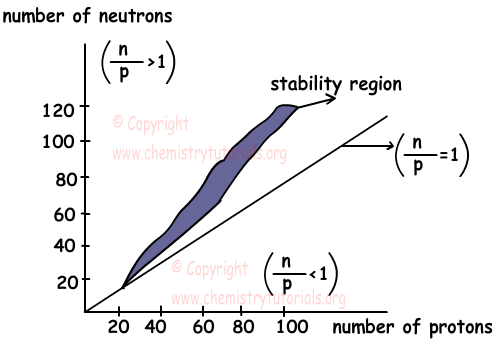Physical and chemical changes do not change structure of nucleus. On the contrary, nuclear chemistry or radioactivity deals with changes in the structure of nucleus. There are protons and neutrons in nucleus of atoms. Protons are positively charged and neutrons are neutral particles. Since same particles repel each other, protons repel each other. Neutrons placed between protons and decrease repulsion force between protons. Ratio between number of protons and neutrons in nucleus shows whether atom is stable or unstable. If;

n0/p+≈1 then atom is stable

n0/p+<1 or n0/p+>1,5 nucleus of atoms are unstable and we call these atoms radioactive elements.

Unstable atoms do some nuclear reactions like radiation or decay and become stable atoms. We can explain radioactivity under two titles, natural nuclear reactions and artificial nuclear reactions. In natural reactions, unstable atoms do radiation and become stable atoms. However, in artificial reactions, unstable atoms can be turn in to stable atoms artificially.

Example: Find whether 2040Ca is stable or not.

Sum of number of protons and neutrons gives us mass number (shown in left top corner of element).

n+p=Mass Number

20+n=40

n=20

where n is number of neutrons and p is number of protons.Thus ratio between n and p is;

n0/p+=20/20=1

Since ratio is equal to 1, 2040Ca is stable atom.

Example: Find whether 92232U is stable or not.

n+p=Mass Number

92+n=232

n=140

where n is number of neutrons and p is number of protons.Thus ratio between n and p is;

n0/p+=140/92=1,6

Since ratio is greater than 1, 92232U has unstable nucleus and it is radioactive element.

• Nucleus of atom consists of protons and neutrons. Energy that keeps them together is called binding energy. If this energy is high, then atom is more stable.
• If a compound is radioactive, at least one of the elements of this compound is radioactive.
• Radioactivity of atoms does not depend on, temperature, pressure, light, electron transfer etc. (any physical or chemical changes)

Example: K, L, M and N elements form compounds KL, K2N and KM. If KL and K2N are radioactive and KM is not radioactive compound, find whether the following compounds are radioactive or not.

I. K2

II. K2L

III. N2M

IV. KN

Solution:

If a compound is radioactive, at least one of the elements of this compound must be radioactive. Since KM is not radioactive, K and M are not radioactive elements. If K2N and KL are radioactive then N and L must be radioactive elements.

K2, is not radioactive but K2L, N2M and KN are radioactive compounds because of radioactive element N.

Differences between Chemical Reactions and Nuclear Reactions: (C.R: chemical Reaction, N.R: Nuclear Reaction)

• In chemical reactions, atoms are organized by breaking chemical bonds and forming new ones. On the contrary, elements or isotopes of elements can turn into other elements in nuclear reactions.
• In C.R only valence electrons play role in breaking and forming bonds, however in N.R protons, neutrons and electrons play role.
• IN C.R. types of atoms are conserved but in N.R, types of atoms can be changed.
• Mass is conserved in C.R. but mass is not conserved in N.R.

Graph given below shows stability of nucleus;• Stable nucleus having atomic number between 1<Atomic mass<20 have ratio n/p≈1, nucleus in this region has equal number of protons and neutrons to become stable.
• Stable nucleus having atomic number between 20<Atomic mass <83 has ratio n/p>1. In this region, number of protons increase and repulsion between them also increases. To balance this force number of neutrons must also increase.
• Nucleus having atomic number higher than 83 has great number of protons and repulsion force between protons. Since the amount of force is too high, number of neutrons can not balance them and nucleus stays unstable. Thus, we can say that nuclei having atomic number greater than 83 are unstable.

Nuclear Chemistry (Radioactivity) Exams and  Problem Solutions

Related